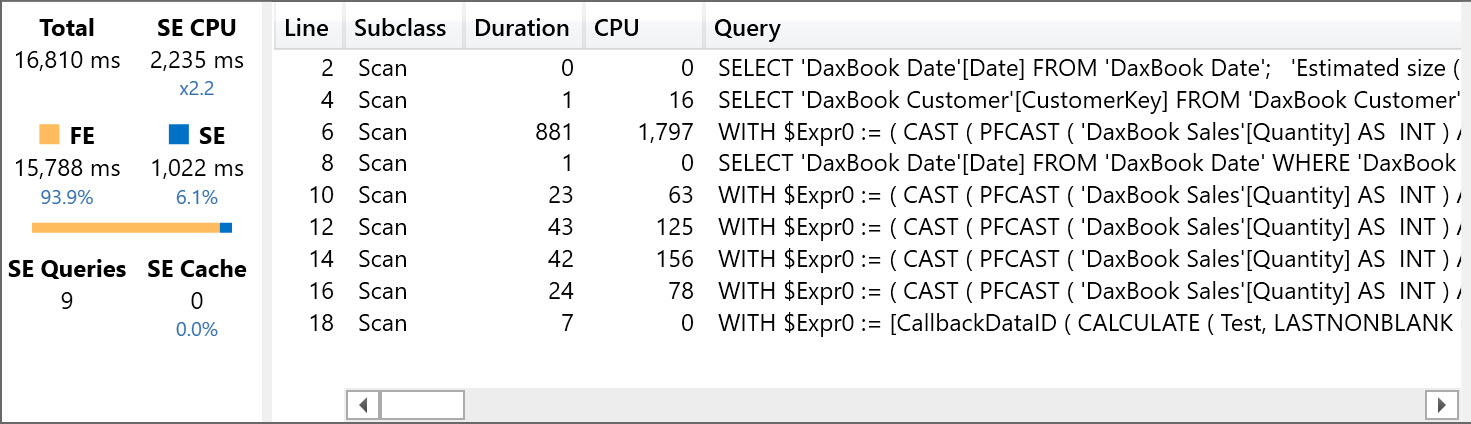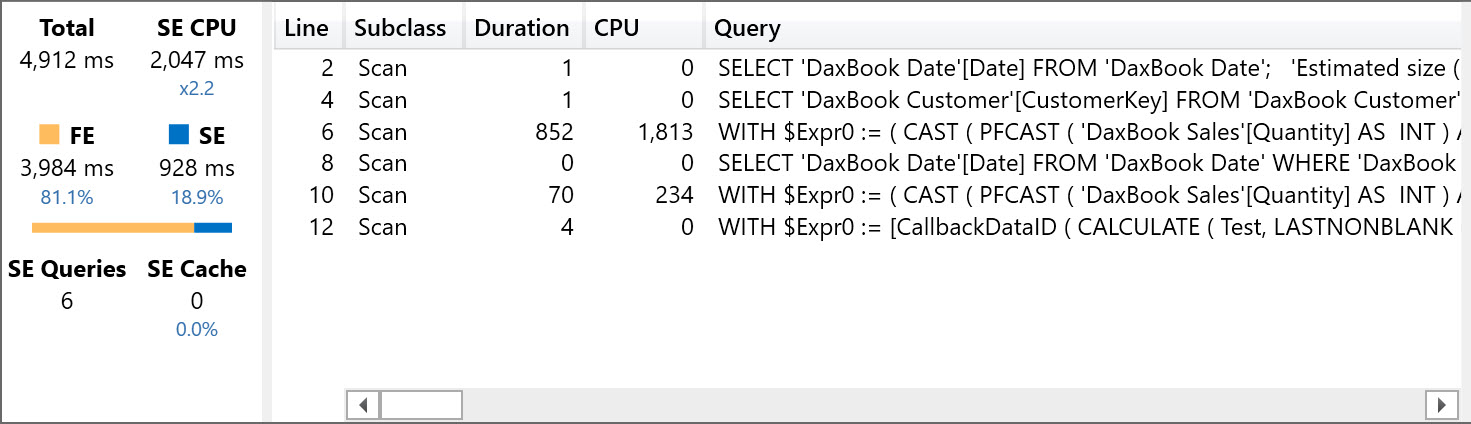In DAX a measure is always a CALCULATE statement. When the expression of a measure references other measures, these nested CALCULATE calls might require a separate calculation or might be merged into a single one. For example, consider this measures’ definition.

```Revenues := SUMX ( Sales, Sales[Sales Line Amount] )
Costs := SUMX ( Sales, Sales[Sales Line Cost] )
Profit := [Revenues] - [Costs]
```

In this case, the calculation of Profit requires a single storage engine operation to be completed in Excel 2016, Analysis Services 2016, or Power BI. In former versions of the DAX Engine (Excel 2010/2013 and Analysis Services 2012/2014), the same expression required two distinct storage engine operations to be completed. The more recent versions of these engines improved the scalability by creating a single request to the storage engine when there are requests of aggregations of different columns with the same filters. Thus, the previous example would generate a single storage engine query instead of two. However, this is possible only when you have different measures (or CALCULATE functions) aggregating columns from the same table and with the same filters. If you have different filters, then you will obtain different sequential calls to the storage engine, resulting in a performance bottleneck for response time.

For example, consider the following query that defines a measure named Test, which sums 4 measures aggregating the year-to-date of Sales Amount of four different product categories. Please note that the year-to-date calculation is required to create a longer and easier to measure execution time.

```DEFINE
MEASURE Sales[Sales Amount] =
SUMX ( Sales, Sales[Quantity] * Sales[Net Price] )
MEASURE Sales[Audio] =
CALCULATE (
[Sales Amount],
'Product Category'[Category] = "Audio",
DATESYTD ( 'Date'[Date] )
)
MEASURE Sales[TV and Video] =
CALCULATE (
[Sales Amount],
'Product Category'[Category] = "TV and Video",
DATESYTD ( 'Date'[Date] )
)
MEASURE Sales[Computers] =
CALCULATE (
[Sales Amount],
'Product Category'[Category] = "Computers",
DATESYTD ( 'Date'[Date] )
)
MEASURE Sales[Cell phones] =
CALCULATE (
[Sales Amount],
'Product Category'[Category] = "Cell phones",
DATESYTD ( 'Date'[Date] )
)
MEASURE Sales[Test] = [Audio] + [TV and Video]
+ [Computers]
+ [Cell phones]
EVALUATE
ROW (
"Test", SUMX (
Customer,
CALCULATE (
[Test],
LASTNONBLANK ( 'Date'[Date], [Sales Amount] )
)
)
)
```

The final SUMX in the query executes the measure Test for each customer, considering only the last day available for each customer. The reason of this loop is just to stress the Test measure and inflate the overall completion time. The query completes in 15 seconds on the hardware I used for the test. You can repeat the measure on your hardware by downloading the sample file, opening the Contoso file in Power BI Desktop, connecting DAX Studio to Power BI Desktop and executing the DAX query. You can see the result in the following screenshot.As you see, there are 4 storage engine queries which are executed sequentially by the formula engine. Then, most of the execution time is caused by the formula engine scanning the data caches returned by several storage engine queries. This could be analyzed in more detail in the query plan, but it would be a long job to describe a 588 lines query plan. Thus, let’s try to simplify the concept. The Test measure is defined as follows:

```Sales[Test] := [Audio] + [TV and Video] + [Computers] + [Cell phones]
```

The four measures that are summed are defined with the following template, where corresponds to the category name used as the name of the measures:

```    MEASURE Sales[<category>] =
CALCULATE (
[Sales Amount],
'Product Category'[Category] = "<category> ",
DATESYTD ( 'Date'[Date] )
)
```

The current version (July 2016) of the engine does not optimize the sum of four categories by creating a single operation. Every CALCULATE is executed in an independent way and for this reason we see different storage engine queries, resulting in a larger materialization made by the storage engine and a longer job for the formula engine. As usual, the trick is to push most of the job down to the storage engine, reducing the pressure on the formula engine.
In this case, we want to sum four categories in a single storage engine query. We can obtain that by using this measure:

```    Sales[Test] =
CALCULATE (
[Sales Amount],
DATESYTD ( 'Date'[Date] ),
'Product Category'[Category] = "Audio"
|| 'Product Category'[Category] = "TV and Video"
|| 'Product Category'[Category] = "Computers"
|| 'Product Category'[Category] = "Cell phones"
)
```

A single CALCULATE statement with a single filter over the ‘Product Category'[Category] column is the way to go. In order to keep the compatibility with other measures returning the value for a single category, you can run this query, which returns the same result in five seconds, three times faster than the previous one.

```DEFINE
MEASURE Sales[Sales Amount] =
SUMX ( Sales, Sales[Quantity] * Sales[Net Price] )
MEASURE Sales[Sales Amount YTD] =
CALCULATE (
[Sales Amount],
DATESYTD ( 'Date'[Date] )
)
MEASURE Sales[Audio] =
CALCULATE (
[Sales Amount YTD],
'Product Category'[Category] = "Audio"
)
MEASURE Sales[TV and Video] =
CALCULATE (
[Sales Amount YTD],
'Product Category'[Category] = "TV and Video"
)
MEASURE Sales[Computers] =
CALCULATE (
[Sales Amount YTD],
'Product Category'[Category] = "Computers"
)
MEASURE Sales[Cell phones] =
CALCULATE (
[Sales Amount YTD],
'Product Category'[Category] = "Cell phones"
)
MEASURE Sales[Test] =
CALCULATE (
[Sales Amount YTD],
'Product Category'[Category] = "Audio"
|| 'Product Category'[Category] = "TV and Video"
|| 'Product Category'[Category] = "Computers"
|| 'Product Category'[Category] = "Cell phones"
)
EVALUATE
ROW (
"Test", AVERAGEX (
Customer,
CALCULATE (
[Test],
LASTNONBLANK ( 'Date'[Date], [Sales Amount] )
)
)
)
```

The first gain is visible in the storage engine queries: one of those replaced four queries that we have seen in the previous test. The duration improvement at the storage engine level is less than 40%, but the reduced materialization produced by a single query reduced the pressure on the formula by more than 70%.I have seen this pattern as a common practice in P&L models, where you have an Account table in the data source, and you need measures that only consider particular accounts. A typical example is something like this:

```[Revenues] := [Sales] + [Royalties]

[Sales] :=
CALCULATE (
SUM ( Movements[Amount] ),
Account[Level1] = "Sales"
)

[Royalties] :=
CALCULATE (
SUM ( Movements[Amount] ),
Account[Level1] = "Royalties"
)
```

The sum of different accounts should be obtained through a single SUM that aggregates all the accounts that can share the same sign. If you have to subtract accounts, you should consolidate all the accounts with the same sign in a single measure, and then apply the difference between these measures. For example, instead of doing this:

```[ComplexAccount] := [A1] + [A2] - [B1] - [B2]
```

You should write:

```[A] := [A1] + [A2]
[B] := [B1] + [B2]
[ComplexAccount] := [A] - [B]
```

And then optimize [A] and [B] using a single CALCULATE statement that filters all the accounts you want to sum in that intermediate calculation.

One final warning: this optimization might become unnecessary in the future in case the DAX engine will improve the query plan generated in this case. Thus, you should always verify that the optimization actually provides a performance advantage for your model, and this optimization should be reconsidered when you upgrade the version of the DAX engine you use (Power Pivot, Power BI, or SSAS Tabular).

You can download the demo files (queries that you can run with DAX Studio over a Contoso database) from the link below. You can also download the Contoso.pbix file (~400MB) from The Definitive Guide to DAX – Companion content.